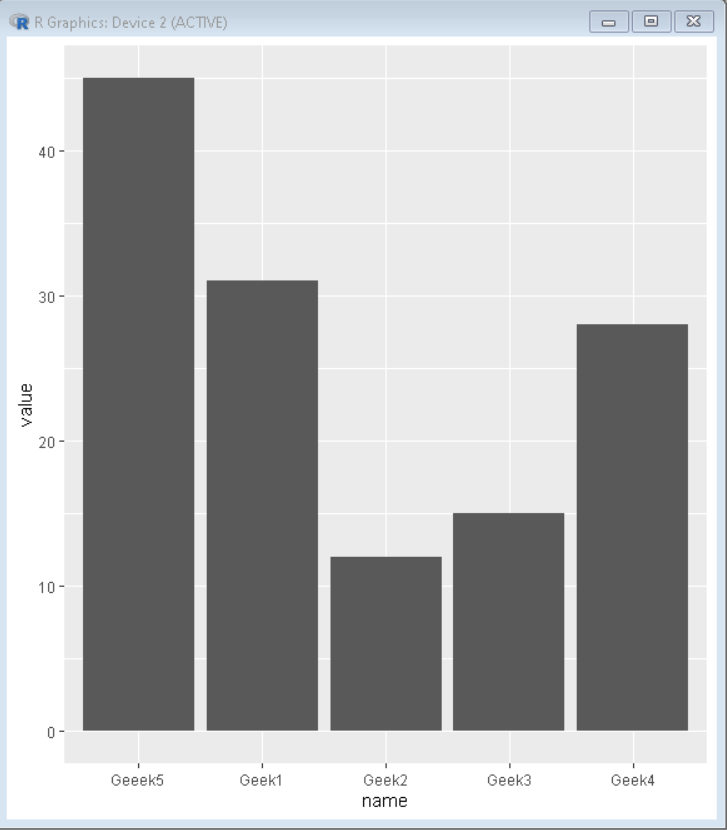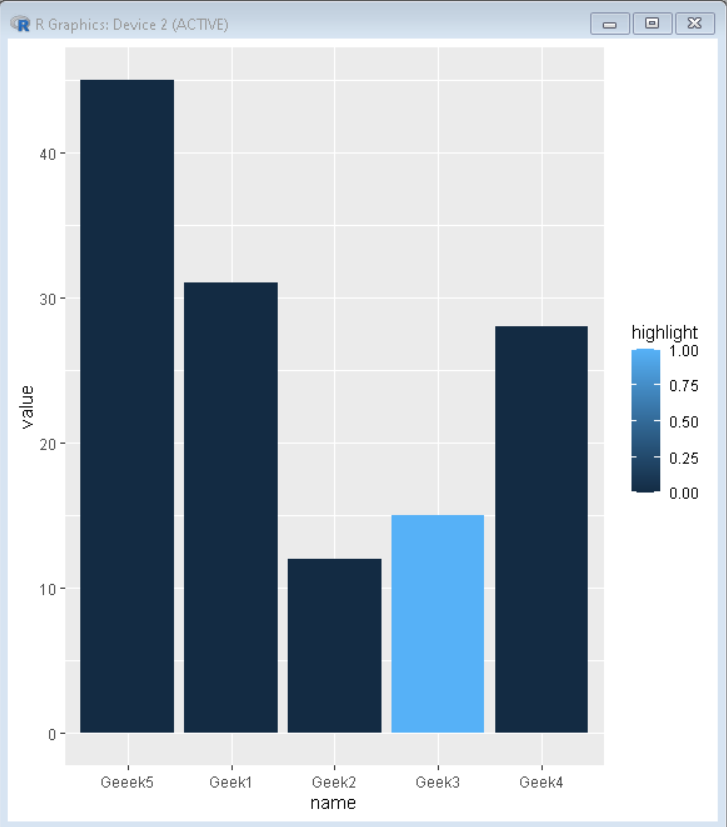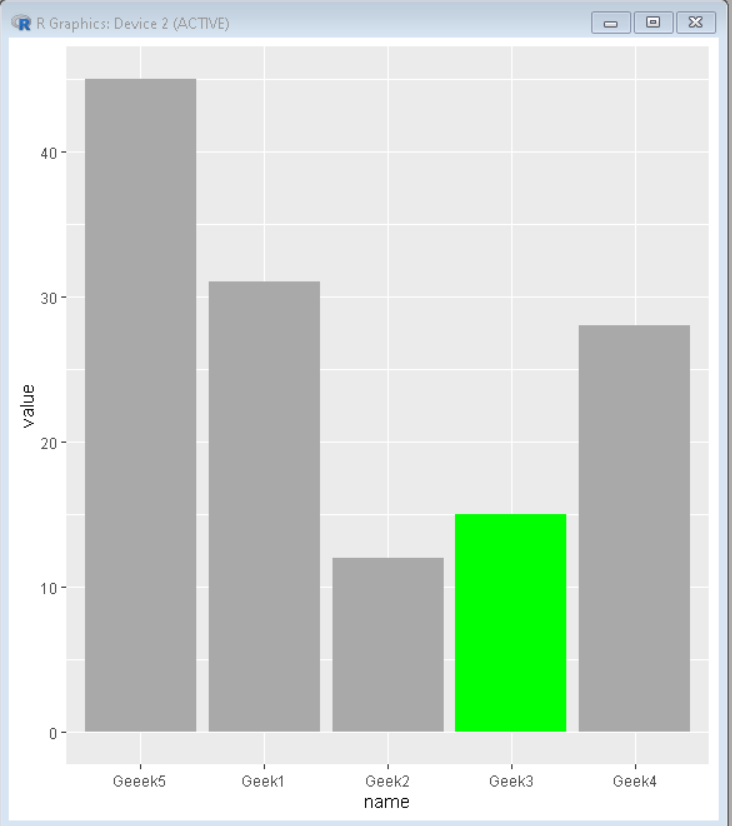# How to Highlight a Bar in Barplot in R?

• Last Updated : 18 Oct, 2021

In this article, we will discuss how to highlight a bar in barplot in R Programming language.

First, let’s create a basic barplot with no bars highlighted using ggplot2.

## R

 `# Create sample data``set.seed``(5642)                            ``sample_data <- ``data.frame``(name = ``c``(``"Geek1"``,``"Geek2"``,``                                   ``"Geek3"``,``"Geek4"``,``                                   ``"Geeek5"``) ,``                          ``value = ``c``(31,12,15,28,45),``                         ``highlight= ``c``(0,0,1,0,0) )``# Load ggplot2 package``library``(``"ggplot2"``)` `# Create bar plot``plot<-``ggplot``(sample_data,``             ``aes``(name,value)) +``        ``geom_bar``(stat = ``"identity"``)``plot`

Output:## Highlighting bar in barplot

To create automated color in bars we use the fill property of the ggplot2 plot. We use a vector with the same value for all the bars except the one that needs to be highlighted.

## R

 `# Create sample data``set.seed``(5642)                            ``sample_data <- ``data.frame``(name = ``c``(``"Geek1"``,``"Geek2"``,``                                   ``"Geek3"``,``"Geek4"``,``                                   ``"Geeek5"``) ,``                          ``value = ``c``(31,12,15,28,45),``                         ``highlight= ``c``(0,0,1,0,0) )``# Load ggplot2 package``library``(``"ggplot2"``)` `# Create bar plot``plot<-``ggplot``(sample_data,``             ``aes``(name, value, fill=highlight)) +``        ``geom_bar``(stat = ``"identity"``)``plot`

Output:## Highlighting specific bar in barplot

To create manual color in bars we use the fill property of the ggplot2 plot with scale_fill_manual() function. We use a vector with the same value for all the bars except the one that needs to be highlighted and then we define a color for each value so every bar is colored according to the value specified to it:

## R

 `# Create sample data``set.seed``(5642)                            ``sample_data <- ``data.frame``(name = ``c``(``"Geek1"``,``"Geek2"``,``                                   ``"Geek3"``,``"Geek4"``,``                                   ``"Geeek5"``) ,``                          ``value = ``c``(31,12,15,28,45),``                         ``highlight= ``c``(``"0"``,``"0"``,``"1"``,``"0"``,``"0"``) )``# Load ggplot2 package``library``(``"ggplot2"``)` `# Create bar plot with highlighted bar``plot<-``ggplot``(sample_data,``             ``aes``(name,value,fill=highlight)) +``        ``geom_bar``(stat = ``"identity"``)+``        ``scale_fill_manual``( values = ``c``( ``"1"``=``"green"``, ``"0"``=``"darkgray"` `),``                          ``guide = ``FALSE` `)``plot`

Output:My Personal Notes arrow_drop_up